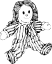## Year 5 - Multiplication

### Choose correct answer(s) from given choice

1)The largest factor of any number is :
 a. Ten b. The number itself c. One d. Hundred
2)Jeff bought 23 books and 15 toys from the market. If the price of each book and toy is Rs. 75 and Rs. 52 respectively, how much money did Jeff pay for the books?
 a. 2505 b. 1725 c. 165 d. 780
3)Mark arranged his toy dolls in 5 rows with 7 dolls in each row as shown below
dollsWhich expression can be used to find the total number of toy dolls
 a. 7 � 5 b. 5 x 7 c. 5 + 7 d. 5+5+5+5+5
4)At a farm, a crate of fruits can hold 26 pears. If a farm worker can fill 10 crates in a day, and the farm has 24 farm workers, how many pears can be packed into crates in 11 days?
 a. 68680 b. 68640 c. 6239 d. 68652
5)If a Publisher produces 11008 books a day, how many books are made in a leap year:
 a. 4028928 b. 4039936 c. 572416 d. 4017920

### Fill in the blanks

6)Solve the following
 5395 X ( 100 + 50 + ) = 852410 438 X ( 500 + 10 + ) = 225132 5352 X ( 900 + + 2 ) = 5041584 8718 X ( + 20 + 9 ) = 2868222
 7) In a hotel, there are 149 rooms. If the one night rent of each room is Rs. 6710, the daily income of the hotel assuming all rooms are occupied is Rs.
 8) If a train travels 150 Km in one hour, then it cover a distance of Km in the month of January if it travels continuously.
9)Find the product of following
 9 6 8 1 0 8 X 6 1 5 9
=
 1 9 0 7 2 9 X 7 2 0 7
=
 10) The number 500 time is 50000
Back to top